# Loan

Apply for a $59000 loan, the loan repayment period is 8 years, the interest rate 7%. How much should I pay for every month (or every year if paid yearly). Example is for practise geometric progression and/or periodic payment for an annuity. Result m = 804.39 USD y = 9880.6 USD #### Solution: $i_1 = 7/100/12 = 0.006 \ \\ r_1 = (1 + i_1)^{ 12\cdot 8 } = 1.776 \ \\ m = 59000 \cdot \dfrac{ i_1 r_1}{ r_1 -1 } = 59000 \cdot \dfrac{ 0.006 \cdot 1.776}{ 1.776 -1 } = 804.39 \ \text { USD }$ $i_2 = 7/100 = 0.07 \ \\ r_2 = (1 + i_2)^{ 8 } = 1.718 \ \\ y = 59000 \cdot \dfrac{ i_2 r_2}{ r_2 -1 } = 59000 \cdot \dfrac{ 0.07 \cdot 1.718}{ 1.718 -1 } = 9880.6 \ \text { USD }$ Leave us a comment of this math problem and its solution (i.e. if it is still somewhat unclear...): Showing 0 comments:Be the first to comment!#### Following knowledge from mathematics are needed to solve this word math problem: ## Next similar math problems: 1. Profitable bank deposit 2012Calculate the value of what money lose creditor with a deposit € 9500 for 4 years if the entire duration are interest 2.6% p.a. and tax on interest is 19% and annual inflation is 3.7% (Calculate what you will lose if you leave money lying idle at negative 2. Compound interest 3After 8 years, what is the total amount of a compound interest investment of$25,000 at 3% interest, compounded quarterly? (interest is now dream - in the year 2019)
3. InflationOnce upon a time, tsar owned a money printer and printed and printed. The result of printing money prices went up,in the first year 3.9 %, in the second 6%, in the third 4.7% and in the fourth 5.5%. Then tsar was failed in election. Calculate the aver
4. Negative percentage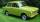In 2004, the company had a loss of 40900 Euros. Two years later he was already in profit 48900 Eur. Calculate what percentage of the company increased profits in this two years.
5. Reducing balance method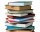A company buys an item having a useful life of 10 years for 1,000,000. If the company depreciates the item by the reducing balance method, a. Determine the depreciation for the first year. b. Estimate the depreciation for the second and third years. c..
6. VAT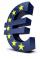John paid 100 Euros in store for purchase. Calculate value added tax (VAT), which paid in purchase, if the VAT rate is 20%.
7. Rates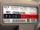When gas consumption, the consumer may choose one of two rates: rate A - which pays 0.4 € per 1 m3 of gas a flat monthly fee of 3.9 € (regardless of consumption) rate B - which pays 0.359 € per 1 m3 of gas a flat monthly fee of 12.5 € From what monthl
8. Engineer KažimírThe difference between politicians-demagogues and reasonable person with at least primary education beautifully illustrated by the TV show example. "Engineer" Kažimír says that during their tenure there was a large decline in the price of natural gas, pri
9. Before yesterdayHe merchant adds a sale sign in his shop window to the showed pair of shoes in the morning: "Today by p% cheaper than yesterday. " After a while, however, he decided that the sign saying: "Today 62.5% cheaper than the day before yesterday". Determine the n
10. Discount and pricesAfter a 29% discount, the new product price was € 426. What was the original price of the product?
11. Car downSarah buys a car costing $12,500. It depreciates in value by 8% in the first year, 10% the second year and 5% in the third year. Calculate the value of a car after the third year. 12. BonusGross wage was 527 EUR including 16% bonus. How many EUR were bonuses? 13. DiscountLadies sweater was twice discounted. First by 11%, then by 11% of the new price. Its final price was 100 €. Determine the original price of sweater. 14. Aunt Rose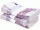Aunt Rose gave$2500 to Mani and Cindy. Mani received \$500 more than Cindy. How mich did Cindy received?
15. StoreOne meter of the textile was discounted by 2 USD. Now 9 m of textile cost as before 8 m. Calculate the old and new price of 1 m of the textile.
16. Cone A2VSurface of cone in the plane is a circular arc with central angle of 126° and area 415 cm2. Calculate the volume of a cone.
17. Sphere slicesCalculate volume and surface of a sphere, if the radii of parallel cuts r1=31 cm, r2=92 cm and its distance v=25 cm.